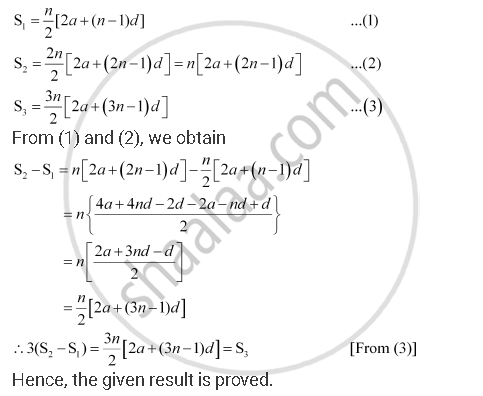Share

# Let the Sum of N, 2n, 3n Terms of an Arithmetic Progression. Be S1, S2 and S3, Respectively, Show that S3 = 3 (S2– S1) - Mathematics

#### Question

Let the sum of n, 2n, 3n terms of an A.P. be S1, S2 and S3, respectively, show that S3 = 3 (S2– S1)

#### Solution

Let a and b be the first term and the common difference of the A.P. respectively.

Therefore,Is there an error in this question or solution?

#### APPEARS IN

NCERT Solution for Mathematics Textbook for Class 11 (2018 (Latest))
Chapter 9: Sequences and Series
Q: 3 | Page no. 199

#### Video TutorialsVIEW ALL 

Solution Let the Sum of N, 2n, 3n Terms of an Arithmetic Progression. Be S1, S2 and S3, Respectively, Show that S3 = 3 (S2– S1) Concept: Arithmetic Progression (A.P.).
S